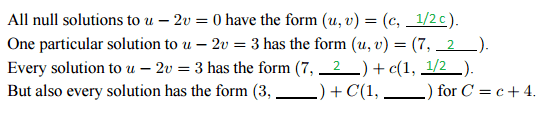# Null solutions

## Homework Statement## The Attempt at a Solution

my answers are in green, and I have no idea how to complete the last part. What is happening?
what does C = c + 4 mean?

Mark44
Mentor

## Homework Statement

View attachment 101989

## The Attempt at a Solution

my answers are in green, and I have no idea how to complete the last part. What is happening?
what does C = c + 4 mean?
Any pair of numbers (c, c/2) is a solution to the equation u - 2v = 0.
This can also be written as c(1, 1/2), with c being an arbitrary constant.
For the equation u - 2v = 3, (7, 2) is a solution, but (7, 2) + c(1, 1/2) is also a solution, for any choice of c. For example, you can verify that if c = 2, (7, 2) + 2(1, 1/2) is a solution. If c = 4, you can verify that (7, 2) + 4(1, 1/2) is a solution.

I'm not sure what you're asking in your last question, but C (upper case) and c (lower case) are different numbers.

Ok, so it means the difference between the two numbers is 4?

so then it should be (3 , -2) + C(1, 1/2) ?

Mark44
Mentor
Ok, so it means the difference between the two numbers is 4?

so then it should be (3 , -2) + C(1, 1/2) ?
Does it work? IOW, does it satisfy u - 2v = 3?

oh, i understand now, its (3, 0)
because 3 - 2(0) = 3

to solve for the last fill in, the best way is to just plug and chug?

so it should be C(1, 1/2)

Mark44
Mentor
What do you get for the last part of the question? In other words what numbers go in the blank spots?
(3, _ ) + C(1, _ )

(3,0) + C(1, 1/2)

Mark44
Mentor
(3,0) + C(1, 1/2)
Sure, that works.

Mark44
Mentor
Earlier you said this:
so then it should be (3 , -2) + C(1, 1/2) ?
I asked you whether your answer satisfied u - 2v = 3. Do you understand why it does or does not?

Earlier you said this:
I asked you whether your answer satisfied u - 2v = 3. Do you understand why it does or does not?
yes, this is much easier than the Yn and Yp stuff. I just need to connect the pieces to the puzzle.

Mark44
Mentor
yes, this is much easier than the Yn and Yp stuff. I just need to connect the pieces to the puzzle.
This problem is a simplified version of the business with a complementary solution and a particular solution. It helps to think in terms of vectors.

This problem has two equations (I have replaced u and v by x and y, respectively, in hopes that it will make things easier to understand):
x - 2y = 0 -- the homogeneous equation
x - 2y = 3 -- the nonhomogeneous equation

The first equation has an infinite number of solutions -- vectors of the form C<1, 1/2>; i.e., any constant multiple of the vector <1, 1/2>. As long as the first coordinate is two times the second coordinate, the vector is a solution to the homogeneous equation. Or, to put in another way, the point (C, C/2) is on the line whose equation is x - 2y = 0, or equivalently, y = (1/2)x.

The second equation also has an infinite number of solutions. Among them are the vectors <3, 0>, <5, 1>, and so on (or the points (3, 0), (5, 1), and so on). You can pick any one of these as a particular solution -- say, <3, 0>

To get to any point on the line x = 2y + 3, you can go from the origin to <3, 0> and then along the line in the proper direction. To get to, say, (7, 2), go to right right from the origin to (3, 0), and then go along the vector from (3, 0) to (7, 2) by the appropriate multiple of <1, 1/2>. In terms of vectors, <7, 2> = <3, 0> + 4<1, 1/2>.

To get to an arbitrary point on the line x = 2y + 3, it's possible to solve for the constant C that does the trick, so that <x, y> = <3, 0> + C<1, 1/2> for an appropriate value of C.

This all ties in to differential equations, with this pair as an example:
y' - 2y = 0 (homogeneous)
y' - 2y = 3 (nonhomogeneous)

The solutions to the homogeneous equation (the complementary solutions) are yc(t) = Ae2t, where A is an arbitrary constant.
A solution to the nonhomogeneous equation (a particular solution) is yp(t) = -3/2, which I got by an educated guess.

The general solution to the nonhomogeneous equation is made up of the complementary solutions, plus the particular solution:
y(t) = yp(t) + yc(t) = -3/2 + Ae2t

Because yc(t) is a solution to the homogeneous equation, it must be true that yc' - 2yc = 0, so adding it to the general solution doesn't change the right side of the equation.

##\frac{d}{dt}(y_c(t) + y_p(t)) - 2(y_c(t) + y_p(t) = \frac{d}{dt}(Ae^{2t} - \frac 3 2) - 2(Ae^{2t} - \frac 3 2} = 2Ae^{2t} - 0 - 2Ae^{2t} + 3 = 3##

Thinking about this in terms of vectors, the particular solution gets us up to the space (of functions) that is generated by ##Ae^{2t}##, and the constant A gets us to a particular function in that space.